• Shuffle
Toggle On
Toggle Off
• Alphabetize
Toggle On
Toggle Off
• Front First
Toggle On
Toggle Off
• Both Sides
Toggle On
Toggle Off
Toggle On
Toggle Off
Front

### How to study your flashcards.

Right/Left arrow keys: Navigate between flashcards.right arrow keyleft arrow key

Up/Down arrow keys: Flip the card between the front and back.down keyup key

H key: Show hint (3rd side).h key

A key: Read text to speech.a keyPlay buttonPlay buttonProgress

1/74

Click to flip

### 74 Cards in this Set

• Front
• Back
 If a number of equally sized spheres (representing atoms) are placed in a rectangular box with the restriction that they must be in a regular arrangement, what are the two most efficient forms of packing and how are their layers describes respectively?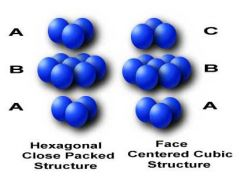Hexagonal close packing (HCP), has ABAB packing. Cubic close packing (CCP), has ABCABC packing. What type of lattice do HCP and CCP lattices have respectively? HCP: primitive lattice (P)CCP: Face-centred subic lattice (FCC) What is the coordination of each sphere for both CCP and HCP structures? 12 HCP and CCP are the most efficient forms of packing known as 'close-packing'. What is another form of packing?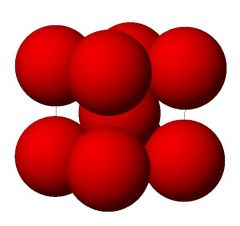Body centred packing (BCC) which has a body-centred cubic lattice. What is important to note about CCP and BCC respectively when considering volume calculations of a single unit cell? In CCP atoms are in contact along the diagonal of the face of the unit cell. Diagonal = 4r.In BCC atoms are touching along body diagonal of unit cell =4r. Calculate the percentage space filled in a CCP lattice: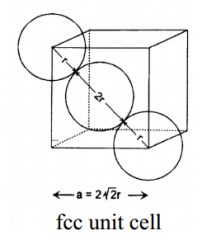As cubic can assume length of all sides = 1.4r² = 1² + 1²r=(√2)/4Volume of a sphere = (4/3)πr³ = 0.185cm³ Number of atoms in unit cell = 4. Total volume of spheres = 0.74cm³. Total volume of cube = 1³ therefore = 74% filled. What can be noted about the percentage space filled in an HCP lattice? Both methods equally efficient, 74% also filled. Calculate the percentage of space filled in a BCC lattice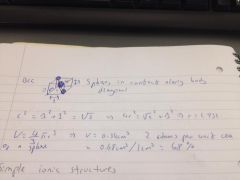Distinguish between tetrahedral and octahedral holes in close-packed structures. how many of each do CCP and HCP have respecitvely?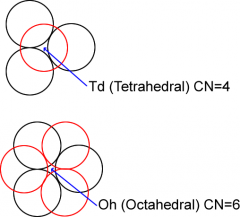Twice as many tetrahedral holes as octahedral. For n anions, there will be 2n tet sites and n oct sitesCCP: 8 tet holes and 4 oct.HCP: 4 tet holes and 2 oct Describe the simple ionic structure of NaCl in terms of the array of the anions, the position of the cations, how many of each atom per unit cell and their respective coordinations. Describe the layering in the strucutre. CCP array of Cl- with Na+ in all octahedral holes. 4 of each ion per unit cell with coordination of 6,6. Ions in alternate layers parallel to {111} planes Describe the simple ionic structure of NiAs in terms of the array of the anions, the position of the cations, how many of each atom per unit cell and their respective coordinations. Describe the layering in the strucutre. HCP array of As atoms with Ni in all octahedral holes. 6,6, coordination with 2 of each atom per unit cell and alternate layers parallel to {001} planes. Describe the simple ionic structure of CdCl2 in terms of the array of the anions, the position of the cations, how many of each atom per unit cell and their respective coordinations. Describe the layering in the strucutre. CCP array of Cl- anions with Cd2+ cations in half of octahedral holes. 6,3 coordination with 4 Cl- and 2 Cd+ per unit cell, alternate layers parallel to {111} planes. Rhombohedral lattice not cubic. Describe the simple ionic structure of CdI2 in terms of the array of the anions, the position of the cations, how many of each atom per unit cell and their respective coordinations. Describe the layering in the strucutre. HCP array of I- anions with Cd2+ cations in half of octahedral holes. 6,3 coordination with 2I- and 1 Cd2+ per unit cell. Alternate layers with {001} planes. Describe the simple ionic structure of ZnS (zinc blende) in terms of the array of the anions, the position of the cations, how many of each atom per unit cell and their respective coordinations. CCP array of S2- anions with Zn2+ in half of the tetrahedral holes. 4,4 coordination with 4 of each ion per unit cell. Describe the simple ionic structure of diamond in terms of the array of the anions, the position of the cations, how many of each atom per unit cell and their respective coordinations. CCP array of C atoms with more C atoms in half of tetrahedral holes. Coordination is 4,4 with 8 C atoms per unit cell. Describe the simple ionic structure of ZnS (Wurtzite) in terms of the array of the anions, the position of the cations, how many of each atom per unit cell and their respective coordinations. HCP array of S2- anions with Zn2+ in half of tetrahedral holes. Coordination 4,4 and 2 of each atom per unit cell Describe the simple ionic structure of 'hexagonal diamond' in terms of the array of the anions, the position of the cations, how many of each atom per unit cell and their respective coordinations. HCP array of C atoms with more C atoms in half of tetrahedral holes, gives 4,4 coordination and 4 C atoms per unit cell. Describe the simple ionic structure of CaF2 (fluorite) in terms of the array of the anions, the position of the cations, how many of each atom per unit cell and their respective coordinations. CCP array of Ca2+ cations with F- anions in all tetrahedral holes. 8,4 coordination with 8 F- and 4 Ca2+ per unit cell Describe the simple ionic structure of Li2O (anti-fluorite) in terms of the array of the anions, the position of the cations, how many of each atom per unit cell and their respective coordinations. CCP array of O2- anions with Li+ cations in all tetrahedral holes, 8,4 coordination with 8 Li+ and 4 O2- per unit cell Describe the simple ionic structure of TiO2 (rutile) in terms of the array of the anions, the position of the cations, how many of each atom per unit cell and their respective coordinations. HCP array of O2- anions with Ti4+ in half of octahedral holes. 6,3 coordination with 4O2- and 2 Ti4+ per unit cell. Describe the simple ionic structure of CsCl in terms of the array of the anions, the position of the cations, how many of each atom per unit cell and their respective coordinations. Looks body centred cubic but actually primitive cubic array of Cl- anions with Cs+ in the body centre of the unit cell. 8,8 coordination with 1Cs+ and 1 Cl- per unit cell. Describe the simple ionic structure of SrTiO3 (perovskite) in terms of the array of the anions, the position of the cations, how many of each atom per unit cell and their respective coordinations.How else can this be viewed? Primitive cubic structure overall.Ti4+ at cell vertices, Sr2+ at body centre and O2- along cell edges. Sr2+ coordinated to 12 O2- anions Ti4+ coordinated to 6 O2- anionsO2- coordinated to 4 Sr and 4 Ti cations.Can be viewed as TiO6 octahedra with Sr in the intersects. What's a simple way of finding number of octahedral holes filled for an anionic array? How can this be used to find number of tetrahedral holes filled? Number of octahedral holes filled = Number of cations / number of anions.Number of tetrahedral holes = octahedral/2 Calculate the density of CsCl with an NaCl type lattice given that the internuclear distance is 3.47 Å Density = Mr * z / V * NA z = number of formula units, v = volume, NA = Avagadros. NaCl has a CCP structure for each ion, with an arrangement of Na+ Cl- Na+ along a unit cell edge. Length of cell there is 2(3.47 Å). Volume = Density = 168.35g/mol * 4 / 0.602 x (6.94Å)³ = 3.35gcm-³ Calculate the density of CsCl with a primitive lattice given that the internuclear distance is 3.56 Å Density = Mr * z / V * NA Diagonal length of body (r) = 2(3.56).Diagonal of base of cube can be found in terms of r: 2a² = r² so r=√2aCan now use r in terms of a and the length of the body diagonal to find a.7.12² = a² r² therefore 50.69=a² + (√2a)²3a² = 50.69 so a=√(50.69/3) = 4.11ÅDensity = 168.35g/mol * 1 / 0.602 x (4.11Å)³ = 4.03g/cm³ Explain the thermodynamics of defect formation Defect formation enthalpically unfavourable but this is counteracted by the associuated increase in entropy. However, as the concentration of defects increases enthalpic cost outweighs entropic gain. What is an intrinsic defect? A defect due to thermodynamic effects What is an extrinsic defect? Defects due to imperfections in stoichiometry Define a point defect Local distortions and localised charge imbalance Define an extended defect When defect sites cluster or accumulate into lines or planes Name 3 important intrinsic defects 1) Schotty defect 2) Frenkel defect 3) Interchange defect What is a Schotty defect and what is it most common for? A pair of cation/anion vaccancies with no change in the stoichiometry. Common for alkali/metal halide compounds. What is a Frenkel defect and for which compounds is it most common? An atom or ion is displaced into an interstitial site with no change in stoichiometry. Most common for AgCl and fluorite/antifluorite conformations. Interstitial sites can sometimes attract each other and form clusters. What is an interchange defect and for which compounds does it usually occur? An interchange of two different atom sites, no change in stoichiometry and common for alloys of Au-Cu Name two forms of extrinsic defects: 1) Doping 2) Crystal impurities Describe the cause of crystal impurity Perfect purity is generally unattainable for crystals of any significant size, impurity atoms or ions incorporated during crystal growth. What is doping? Give a common example The deliberate introduction of impurities to change the properties of a compound. For example changing the electrical/optical properties of Si by introducing B and As atoms. When is a doped compound considered a solid solution? When dopant concentrations are higher than 1% What are colour centres? For which compounds are they most common? Colour centres are extrinsic defects that give rise to changes in optical properties (IR, UV or vis absorption). Most common for alkali metal compounds. What is an F-centre colour defect, how can it be induced for NaCl and what does it result in? Some anion sites are replaced by e-. E.g. by heating NaCl in Na vapour some Cl- sites are replaced by e- . The electron behaves like a particle in a box with quantized energy levels accessible by absorption in the visible part of the spectrum. NaCl with F-centre colour defects appears yellow. Name another compound that is analagous with NaCl in terms of F-centre defects and what colour it appears? KCl, appears violet What is an H-centre colour defect for NaCl? A Cl2- anion fills an anion site What is a V-centre colour defect for NaCl? A Cl2- anion fills a pair of anion sites What is band theory? A description of bonding and conduction in solids What can be noted about the difference in energy between bonding and anti-bonding orbitals for strong and weak interactions? Larger difference in energy between bonding and antibonding for stronger interactions that weaker interactions For a simple solid with only one atom type, what bands would appear? Separate bands resulting from each set of atomic orbitals, i.e. an s-band, a p-band, a d-band etc. What effects the width of the bands? The strength of the interaction, i.e. a strong interaction gives a wide band and a weak interaction a narrow band. Some bands tend to overlap in energy, e.g. 3s and 3p, whilst there are also energy gaps where no orbitals are present. What are these known as?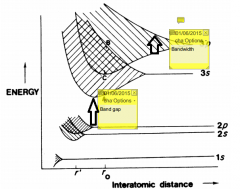Band gaps. Energy levels are filled from lowest to highest bands as for molecular orbitals. What is the highest occupied energy level (at absolute zero) known as? The Fermi level At temperatures above absolute zero what can be noted about the population of the bands? They follow Fermi-Dirac distribution What are phonons? Lattice vibrations in particle form, increase with temperature. What are the properties of metallic conductors? Give some examples: High conductivity with a significant proportion of valence electrons delocalised. Conductivity decreases with temperature due to resistance from phonon-electron collisions. Includes metals, alloys, sulfides What are the properties of semiconductors? Give some examples: Moderate conductivityConductivity increases with temperatureConductivity can be modified by impuritiesIncludes transition metals, Si, Ge What are the properties of insulators? Give some examples: Very low conductivity, conductivity increases with temperature, valence electrons tightly bound, includes many solid covalent networks and ionic solids. What are the properties of ionic conductors? Give some examples: Moderate conductivity, rigid framework with one set of mobile ions. Vacant sites and atoms in interstitial sites increase ion mobility and low energy barriers for high ionic conductivity. Most common in doped materials. Conductivity increases with temperature due to higher concentration of defects. What are the properties of ionic conductors? Give some examples: No electrical resistance below critical temperature (as no electron-phonon collisions). Valence shell delocalised, electrons move cooperatively. What are the properties of superconductors? No electrical resistance below critical temperature, as no electron phonon collisions. Valence shell electrons delocalised and electrons move cooperatively. What can be noted about the difference in energy level transition between band theory and molecular orbital theory? Electrons can be readily promoted to higher bands at thermal temperatures. What property of the bands allows metallic conduction? Give an example using Be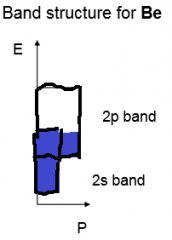Partially filled bands, athe bands must be partially filled. Most metals have partially filled atomic orbitals so partially filled bands, in other cases such as Be the 2s and 2p bands overlap to give partially filled bands and can conduct. Describe the bands of insulators?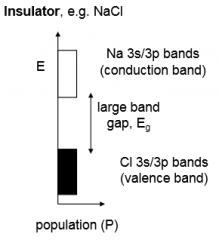They have filled bands and empty bands with large gaps between them, limiting conduction of electrons. Describe the bands of intrinsic semi-conductors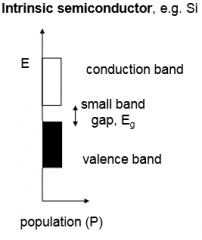They have filled bands and empty bands but small band gaps that allows some conduction due to thermal excitation from the valence band to the conduction band. This can even occur at room temperature to some extent due to Boltzman distribution. Conductivity increases with temperature as increased population of conduction band outweighs increased vibrations. How can charge carriers be viewed? As electrons in the conduction band and as positive holes in the valence band. What is the equation for measuring conductivity? σ = A e^(-Ea/kT) activation energy is approx 0.5E(gap) How can promotion of electrons from valence band to conduction band with larger gaps be induced? Irradiation - photoconductivity. CdS absorbs visible light, used in photocells for solar power How is conduction in extrinsic semi-conductors determined? Semi-conductors are modified by introduction of a doping agent, conductivity generally greater than intrinsic due to decreased band gap. Controlled by the concentration of the dopant. What are P-type semi-conductors? Give an example. Intrinsic semi-conductors where the dopant has one electron fewer than the semiconductor. E.g. introduction of Ga to Si. electron defficient Ga-Si bond not part of valence band, forms discrete acceptor band above it. Electrons from valence band promoted to acceptor band, leaving positive holes to serve as charge carriers. What are N-type semi-conductors? Give an example. Intrinsic semi-conductors where the dopant has one electron more than the semi-conductor. E.g. introduction of As to Si. Extraa electron in energy band below valence band, electrons can be readily promoted into valence band to allow conduction with negative charge carriers. Which type of semi-conductor is best at low and high temps respectively? At low temperature extrinsic higher conductivity, at high temps intrinsic. What property allows solid electrolytes to show very high conductivities? Commeont on their stability Channel structures that permit facile ion mobility. Only stable at high tempertatures. How do superconductors work? Zero electrical resistance below critical temperature as no electron phonon collisions - power transmission without energy loss. Electrons associate into pairs to act cooperatively and travle throughout the structure. 'Cooper' pairs of electrons arise following a local distortion - a second electron is attracted in response. Give an example of a high Tc (critical temp) semi-conductor and state what structure its based on YBa2Cu3O7, based on perovskite ABO3 with oxygen site deficiencies. Comment on the positions of the atoms in YBa2Cu3O7 Y3+ and Ba2+ in body centre with Cu2+/3+ in the cell vertex and O2- along some edges. What is a consequence of the oxygen site deficiencies? How does this result in conductivity? Some Cu ion sites are 2+ and so square pyramidal, and some are 3+ and so square planar. Conductivity due to transfer of electrons between these two structures. How can super conductivity of YBa2Cu3O7 be destroyed? Partial reduction